×#### Thank you for registering.

One of our academic counsellors will contact you within 1 working day.

Click to Chat

1800-1023-196

+91-120-4616500

CART 0

• 0

MY CART (5)

Use Coupon: CART20 and get 20% off on all online Study Material

ITEM
DETAILS
MRP
DISCOUNT
FINAL PRICE
Total Price: Rs.

There are no items in this cart.
Continue Shopping• Complete JEE Main/Advanced Course and Test Series
• OFFERED PRICE: Rs. 15,900
• View Details

```Chapter 15: Linear Inequations – Exercise 15.5

Linear Inequations – Exercise – 15.5 – Q.1

We have,

x + 2y - y ≤ 0

⟹ x + y ≤ 0

Converting the given inequation into equation we obtain, x + y = 0.

Putting y = 0, we get x = 0

Putting x = 0, we get y =0

Putting x = 3, we get y = -3.

We plot these points and join them by a thick line. This lines divider the xy - plane in two parts. To determine the region represented by the given inequality consider the inequality.

So, the region containing the origin is represented by the given inequation as show below: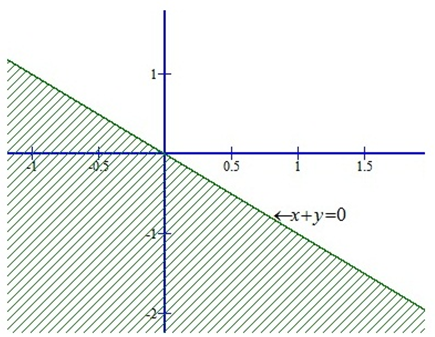This region represents the solution set of the given inequations.

Linear Inequations – Exercise – 15.5 – Q.2

We have,

x + 2y ≥ 6

Converting the inequation into equation, we obtain, x + 2y = 6.

Putting y = 0, we get x = 6

Putting x = 0, we get 2y = 6 ⟹ y = 3

We plot these points and join them by a thick line. This lines divider the xy - plane in two park. To determine the region represented by the given inequality consider the point 0(0, 0).

Putting x = 0 and y = 0 in (i) we get, 0 ≥ 6

It is not possible.

Clearly, 0(0, 0) does not satisfies the inequality.

So, the region represented by the given inequation is the shaded region shown below:Linear Inequations – Exercise – 15.5 – Q.3

We have,

x + 2 ≥ 0 .     ........ (i)

Converting the inequation into equation, we obtain, x = - 2. Clearly, it is a line parallel to y-axis. This line divides the xy - plane in two parts. One part on the LHS of x = -2 and the other on its RHS.

Putting x = 0 in the inequation (i), we get 2 ≥ 0

We find that the point (0, 0) satisfies the inequality. So, the region represented by the given inequation is the shaded region shown below: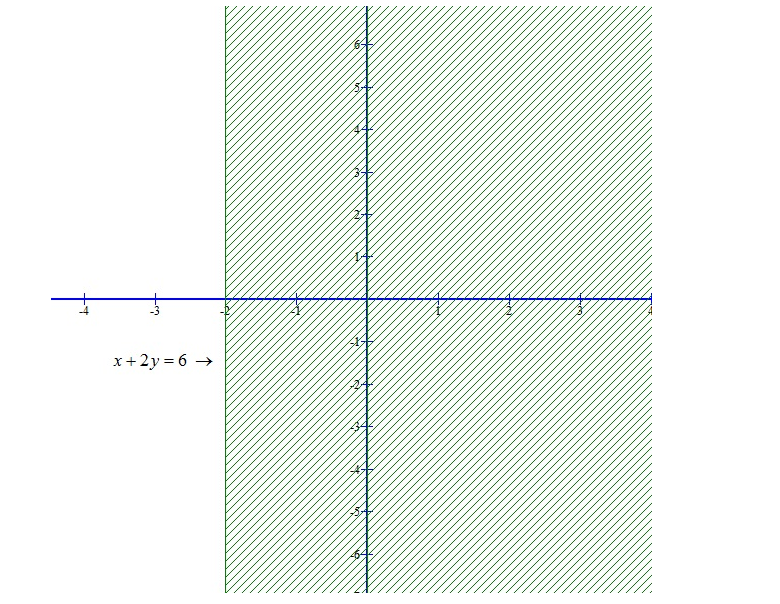Linear Inequations – Exercise – 15.5 – Q.4

We have

x – 2y < 0

Converting the inequation into equation, we obtain,

x < 2y

To determine the region represented by the given inequality consider the point 0(0, 0)

Putting x = 0 and y = 0 in equation we have

0 < 0

It is not possible. Clearly 0(0, 0) does not satisfy the inequality.

So, the region represented by the given in equation is the shaded region shown below:Linear Inequations – Exercise – 15.5 – Q.5

We have,

- 3x +2y ≤ 6       ........ (i)

Converting the given inequation into equation, we obtain, -3x +2y = 6.

Putting x = 0, we get y = 6/2 = 3

Putting y = 0, we get x = -6/3 = - 2

we plot these points and join them by a thick line. This line meets x-axis at (-2, 0) and y-axis at (0, 3). This line divides the xy-plan into two parts. To determine the region represented by the given inequality, consider the point 0(0, 0).

Putting x = 0 and y = 0 in the inequation (i), we get, 0 ≤ 6

Clearly, (0, 0) satisfies the inequality. So the region containing the origin is represented by the given in equation as shown below.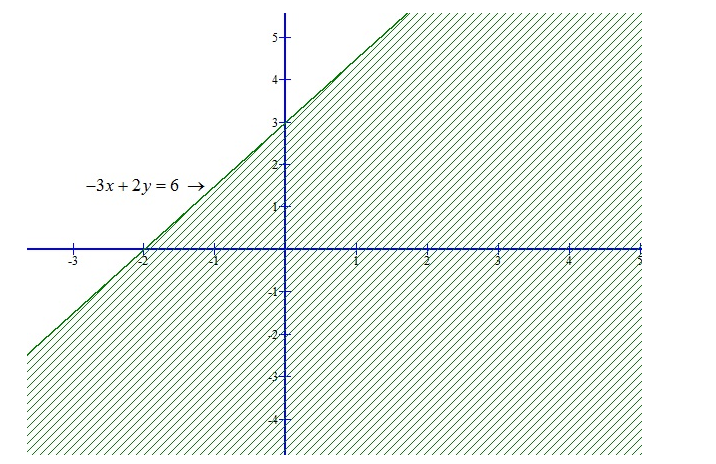Linear Inequations – Exercise – 15.5 – Q.6

We have,

x ≤ 8 - 4y      ..........  (i)

Converting the given in equation into equation, we obtain, x = 8 - 4y.

Putting y = 0, we get x = 8

Putting x = 0, we get y = 8/4 = 2

So, this line meets x-axis at (8, 0) and y-axis at (0, 2).

we plot these points and join them by a thick line. This line divides the xy-plane in two parts. To determine the region represented the given inequality consider the point 0(0, 0).

Putting x = 0 and y = 0 in the inequation (i), we get 0 < 8

Clearly, (0, 0) satisfies the inequality. so, the region containing the origin is represented by the given inequation as shown below: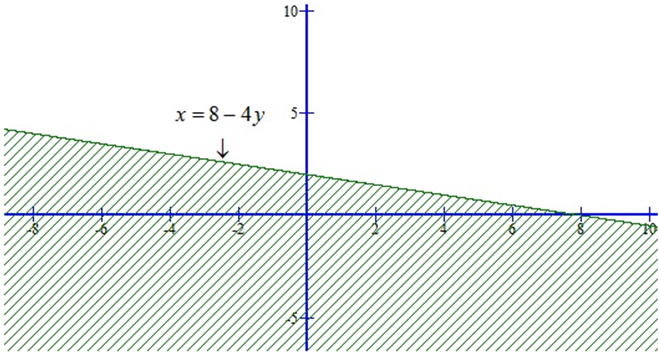Linear Inequations – Exercise – 15.5 – Q.7

We have,

0 ≤ 2x - 5y +10      ........ (i)

Converting the given in equation into equation, we obtain, 2x - 5y + 10 = 0.

Putting x = 0, we get y = -10/-5 = 2

Putting y = 0, we get x = -10/2 = - 5

So, this line meets x-axis at (-5, 0) and y-axis at (0, 2).

we plot these points and join them by a thick line. This line divides the xy-plane in two parts.

To determine the region represented by the given inequality consider the point 0(0, 0).

Putting x = 0 and y = 0 in the inequation (i), we get 0 ≤ 10

Clearly, (0, 0) satisfies the inequality. so, the region containing the origin is represented by the given inequation as shown below: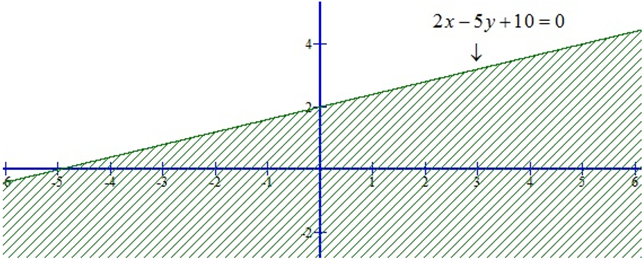Linear Inequations – Exercise – 15.5 – Q.8

We have,

3y ≥ 6 - 2x    ..............  (i)

Converting the given inequation into equation, we obtain, 3y = 6 - 2x.

Putting x = 0, we get y = 6/3 = 2

Putting y = 0, we get x = 6/2 = 3

So, this line meets x-axis at (3, 0) and y-axis at (0, 2).

we plot these points and join them by a thick line. This line divides the xy-plane in two parts. To determine the region represented by the given inequality consider the point 0(0, 0).

Putting x = 0 and y = 0 in the inequation (i), we get 0 ≥ 6 it is not possible.

∴ we find that the point (0,0) does not satisfy the equation 3y ≥ 6 - 2x.

So, the region represented by the given equation is shaded region shown below: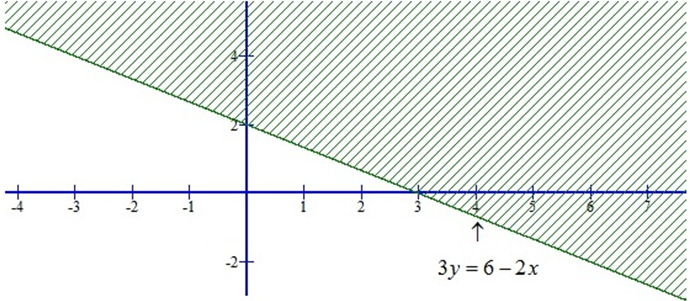```### Course Features

• 728 Video Lectures
• Revision Notes
• Previous Year Papers
• Mind Map
• Study Planner
• NCERT Solutions
• Discussion Forum
• Test paper with Video Solution